# Heptagons - polygons with 7 sides

By Martin McBride, 2022-10-09
Tags: heptagon 7 sided shape regular polygon irregular polygon
Categories: gcse geometryA heptagon is a flat shape with 7 straight sides.

## Regular and irregular heptagons

A regular heptagon is a 7-sided shape where every side is the same length and every corner has the same angle. All regular heptagons have the same shape, like this:An irregular heptagon is a 7 sides shape where not every side and angle are equal. There are many different irregular heptagon shapes, here is an example: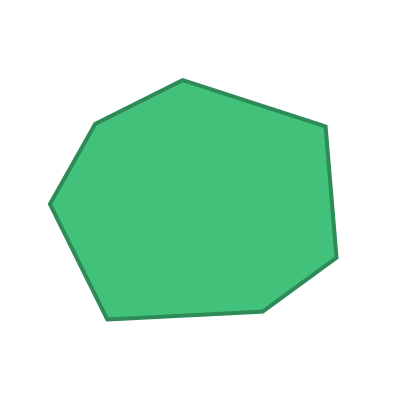## Name

The name heptagon is a combination of the words hepta (derived from the Greek for seven) and gonia (Greek meaning corner).

Heptagons are sometimes called Septagons based on the Latin word septua (meaning seven).

Heptagons are sometimes called 7-gons.

## Interior angles

The interior angles of a heptagon are shown here: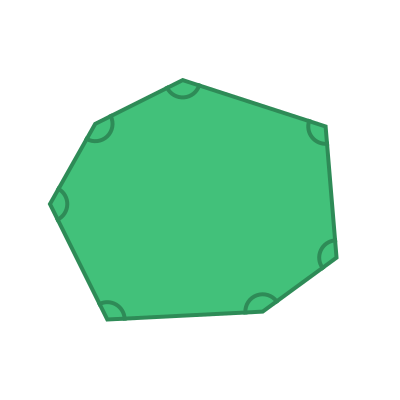The sum of these 7 angles is given by the formula:

sum of interior angles = (n - 2) x 180


Where n is the number of sides. In this case, the number of sides n is 7, so the sum of the interior angles is:

(7 - 2) x 180 = 900 degrees


For a regular heptagon, all the interior angles are equal: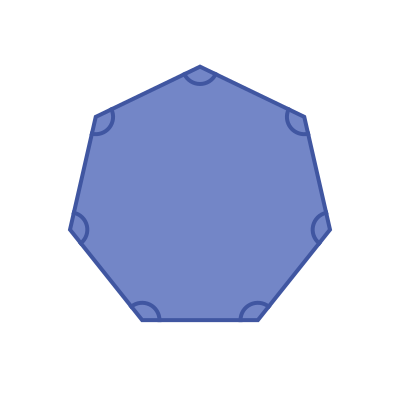This means that the interior angle of a regular heptagon is:

900 / 7 =  128.57 degrees (approximately)


## Exterior angles

The exterior angles of a heptagon are shown here: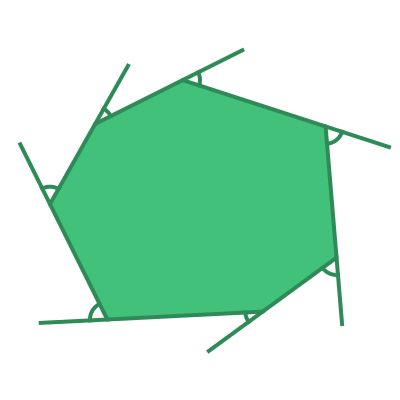The sum of the exterior angles of any polygon is 360 degrees.

For a regular heptagon, all the interior angles are equal: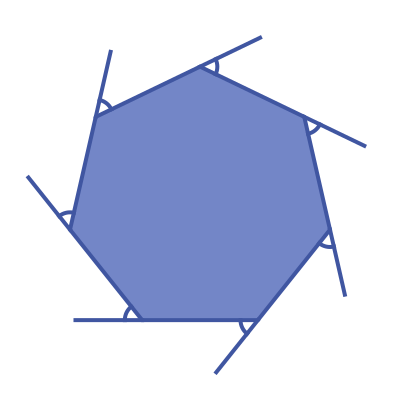This means that the exterior angle of a regular heptagon is:

360 / 7 =  51.43 degrees (approximately)


## Symmetry of regular heptagons

A regular heptagon has 7 lines of symmetry. This means that it can be reflected over each of the 7 grey lines shown here: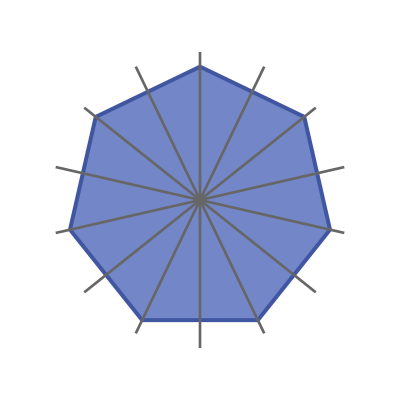A regular heptagon has rotational symmetry of order 7. This means that if it rotated about its centre by a 7th of a full turn, it will map onto itself: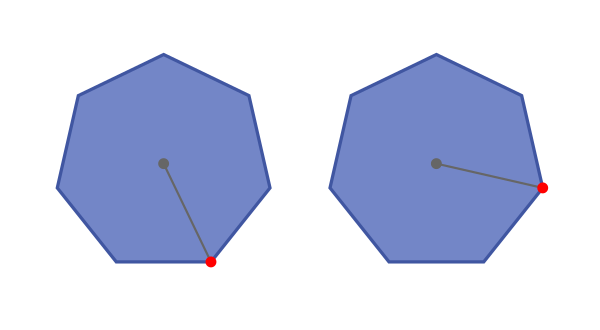## Real-life examples

Some coins are regular heptagons, for example, British 20p and 50p coins.

Many US police badges have a heptogram shape (a heptogram is a str based on the points of a heptogon).